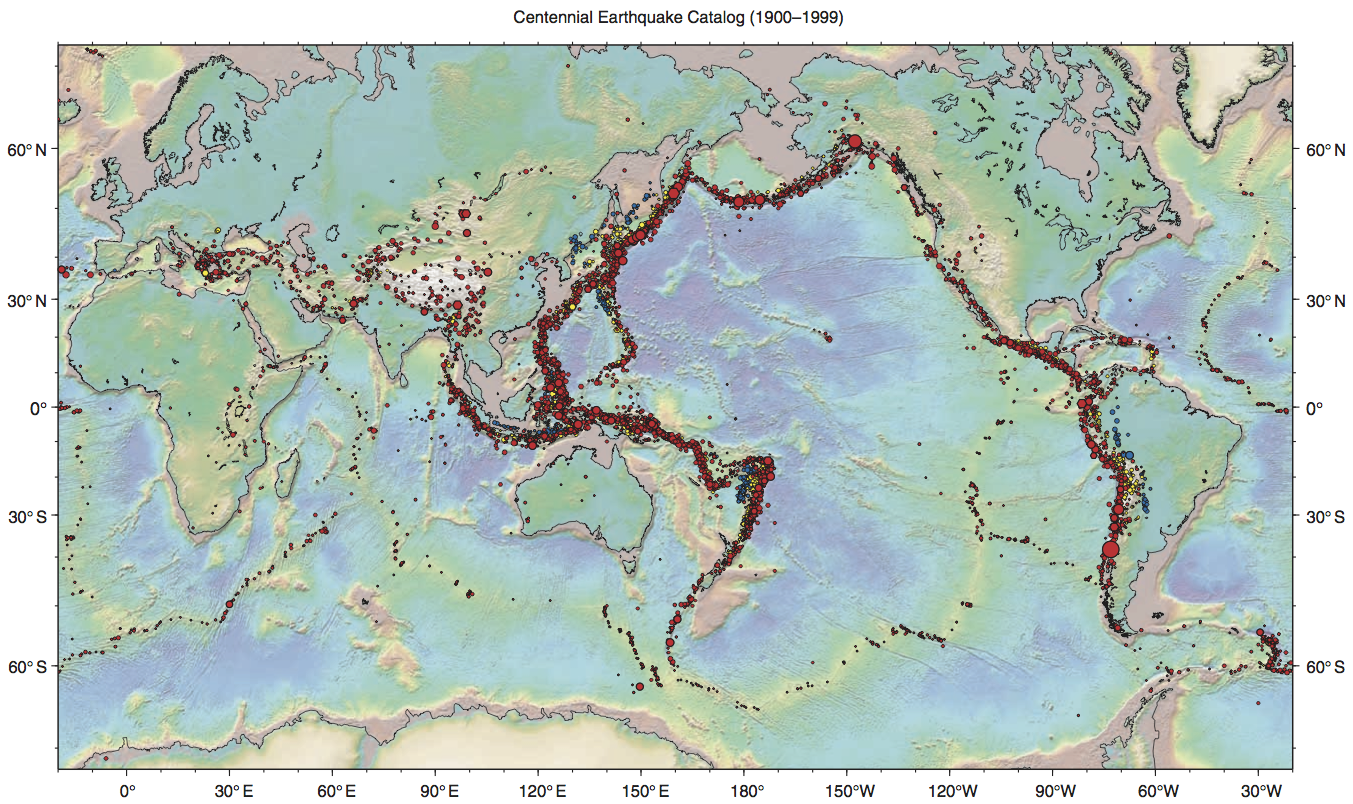December 12, 2016

## Packages and Libraries

• All interesting things in R are done using functions
• We recognize them because they use ()
• Several functions of the same subject are grouped in a package
• To use functions from a package we need to load them using library()
• If the package is not in your computer, you need to use install.packages()

## knitr: a package for Rmarkdown

Knitr is the system that merges R code and Markdown to produce documents that depend on data

It has many functions. We used two of them:

• kable() is a function to produce nicer tables
• The mandatory input is a data.frame
• It is similar to the function pander() from the pander package
• knitr::opts_chunk\$set() to set the default options for each chunk

## Some hints

• remember to use library(knitr) before using any function of the package
• remember that the RMarkdown document is independent of the Console
• Save your document on the X: drive (when using lab computers)

## reshape2: a package to change the shape of data

We will work on reshape2, melt() and aggregate() tomorrow

## We want to make plate 15yr
year
mon
month
day
day
hr
origin hour
min
origin minute
sec
origin second

## Fields

glat
geographic latitude
glon
geographic longitude
dep
focal depth
greg
Flinn-Engdahl geographic region number
ntel
number of observations
mag
magnitude

## Drawing## Hints

The world map can be drawn with the following commands

library(maps)
map('world')

## Energy

The magnitude $$M$$ of an earthquake is related to the released energy $$E$$ (in joules) according to the following expression1: $\log_{10}(E) = 5.24 +1.44 M,$ which is valid for earthquakes over magnitude 5.

Calculate the energy of each earthquake